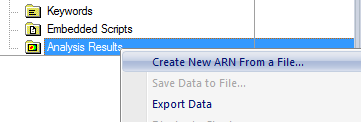# Summing ARNs Using Analysis Surfaces

This knowledge base article discusses how to iterate raytraces and sum the results calculated by an Analysis Surface via a script. A related article also demonstrates how to do this when using Detector Entities.

This method traces in batches and sums the results after each step, saving time when tracing a large number of rays. This is particularly helpful in illumination models where a very large number of rays are required to obtain statistically good data.

This example script sums the Irradiance data and then stores the resultant ARN in the Analysis Results folder on the FRED tree and also as a .fgd file in the current directory.

The script is structured as follows:

```    numloops    = 10
numRays     = 100000        'per source, per loop
analysisID  = FindFullName( "Analysis Surface(s).Analysis 1" )
```

The number of loops, the number of rays (per run, per source), and the Analysis Surface at which the data is to be calculated are defined by the user before the script is run.

Next, the script loops over all entities in the Tree and all sources are reconfigured to use the number of rays defined in the User Settings. Each source is power normalized according to the number of loops so that the total power contributing to the final data set at the end of the iteration loop is consistent with the initial source power settings. Note that it is assumed that each source has Ray Position Type = Random Plane.

```    For i = 0 To GetEntityCount () - 1
If IsSource(i) Then

GetSourcePosGridRandom ( i, semiWidthX, semiWidthY, num, isEllipse )
num = numRays
SetSourcePosGridRandom ( i, semiWidthX, semiWidthY, num, isEllipse )

SetSourcePower i, GetSourcePower( i )/numloops

End If
Next
```

For each iteration of the loop, the rays are traced and an ARN is created. On all but the first iteration, these newly created ARNs are combined with the previously accumulated data using the ARNLinearCombination subroutine and then deleted to save memory.

```    For i = 1 To numloops

EnableTextPrinting False
TraceCreate

If i = 1 Then   'first iteration
Else    'all other iterations

ARNLinearCombination 1, idarn1, 1, idarn2   'summing irradiance data

ARNDelete idarn2
End If

EnableTextPrinting True
Print i & Chr(9) & i * numRays

Next i
```

The accumulated result is saved to the current directory using the ARNWriteToFile subroutine. The file is saved with the name “[numRays] rays - irradiance”.

```    fullfilename    = GetDocDir() & "\" & filename & "-irradiance.fgd"
ARNWriteToFile idarn1, fullfilename, False, False
```

The script creates a new ARN in the Analysis Results folder on the FRED Tree and the fgd file in the current directory. The fgd file can be loaded into the Document at any time by right-clicking the Analysis Results folder on the Tree and selecting " Create New ARN From a File..."Associated FRED Script: SummingARNs---AS.frs

Associated FRED Document: SummingARNsTestFile---AS.frd